# Calculus 2 : Graphing Vectors

## Example Questions

1 3 Next →

### Example Question #21 : Graphing Vectors

In which quadrant does the vector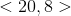terminate from the origin?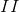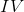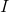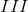Explanation:

The vectorgoes to the rightunits from the origin and then upunits, with terminal point in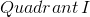### Example Question #22 : Graphing Vectors

In which quadrant does the vector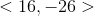terminate from the origin?Explanation:

The vectorgoes to the rightunits from the origin and then downunits, with terminal point in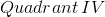### Example Question #23 : Graphing Vectors

In which quadrant does the vector terminate from the origin?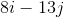Explanation: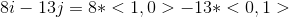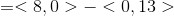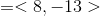The resulting vector terminates in### Example Question #464 : Parametric, Polar, And Vector

In which quadrant does the vector terminate from the origin?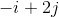Explanation: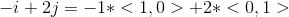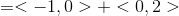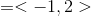The resulting vector terminates in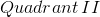### Example Question #25 : Graphing Vectors

In which quadrant does the vector terminate from the origin?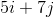Explanation: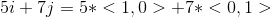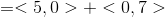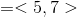The resulting vector terminates in1 3 Next →

### All Calculus 2 Resources